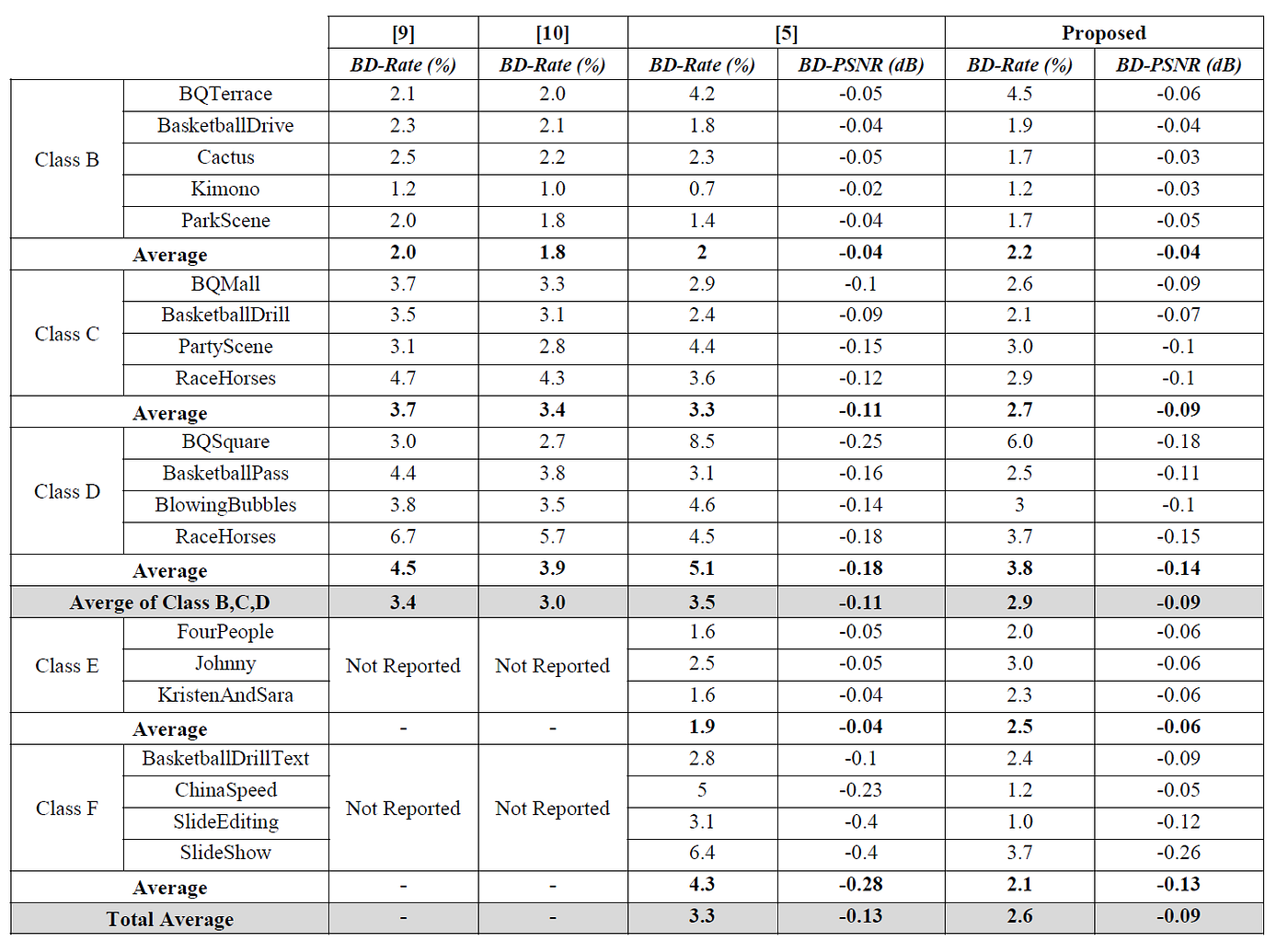# Review: Ibrahim ISM’18 — Neural Networks Based Fractional Pixel Motion Estimation for HEVC (HEVC Inter Prediction)

## Interpolation-Free Method for Fractional Motion Estimation Using Artificial Neural Network (ANN)

In this story, Neural Networks Based Fractional Pixel Motion Estimation for HEVC (Ibrahim ISM’18), by Egypt-Japan University for Science and Technology, Polytechnique Montreal, Zagazig University, and Alexandria University, is reviewed. I read this because I work on video coding research. With the proposed neural network to predict the sub-pel motion vector, interpolation is not needed. This is a paper in 2018 ISM. (Sik-Ho Tsang @ Medium)

# Outline

1. The Use of Fractional Interpolation in Video Coding
2. Proposed Artificial Neural Network (ANN)
3. Experimental Results

# 1. The Use of Fractional Interpolation in Video Coding

• There are correlations between frames for efficient compression using a process called motion compensated prediction (MCP).
• In MCP, for the current block to be coded, the best matching block is searched in previously reconstructed reference frames, and the differences between these two blocks, i.e. residues, are transmitted to the decoder side.
• The positional relationship between current block and its corresponding reference block is represented by a motion vector (MV), which also describes the displacement of these blocks.
• The true frame-to-frame displacements of moving objects may not be integer-pel displacement.
• Fractional-pel precision motion vectors have to be adopted to describe the continuous motion of objects.

Hence, the reference frame/region/block needs to be interpolated in order for Fractional Motion Estimation (FME). Yet, FME process is computationally demanding.

Thus, Artificial Neural Network (ANN) is proposed to predict the fractional position of motion vector so that interpolation is not needed.

# 2. Proposed Artificial Neural Network (ANN)

## 2.1. Architecture

• The inputs are nine matching error values of integer-pixel locations, plus the height and width of the assigned prediction block (PB).
• The FME problem is formulated as a multi-class classification problem, where the output can only be one of 49 points — the center integer point and surrounding 48 pixel locations with quarter precision — as shown in the first figure.
• Authors believed that the most suitable deep learning architecture for that kind of problem is a Fully Connected (FC) ANN.
• The network has a total of 11 inputs, and its output is a Log-Softmax layer with 49 outputs, which predicts the most probable quarter-pixel location for the given inputs.
• Dropout, Batch Normalization (BN), and Entity Embeddings are used.
• Specifically, Entity Embeddings are used for width and height. Entity Embedding is to use a vector to represent an entity. Authors did not provide details about it. But since according to the figure above, 4 neurons are used for either width or height, it maybe an one-hot vector for different size of PB (i.e. 64×64, 32×32, 16×16, and 8×8).
• One ANN was trained for each Quantization Parameter (QP), having a total of four trained ANNs.
• Only two hidden layers were used. The layers consist of 22 and 20 neurons respectively.
• The choice of the number of neurons per layer was mostly arbitrary, where having a smaller number of neurons on the second layer is to reduce the number of computations.

## 2.2. Training

• 6 video sequences are used for training, with mixture of high and low resolutions, and with fast and slow movements.
• Four sets of data were extracted for QP values of {22, 27, 32, 37}, and each set was used to train an independent ANN.
• Each data set was normalized by subtracting the mean and dividing by the standard deviation of each of the inputs.
• For each set, 80% of the data were used for training, and 20% were used for validation.

## 2.3. Computational Cost

• The network, with two hidden layers, requires a total of 1936 additions and 1854 multiplications per prediction, as shown above.
• The only overhead is in terms of memory used to store all four sets of network parameters, and the slight delay of initializing the parameters depending on the used QP, which happens only once per video.

# 3. Experimental ResultsBD-Rate (%) of the Proposed Approach Against the Conventional HEVC HM-16.9
• HM-16.9 with low delay P configuration (Frames are encoded as IPPP…) is used, with fast search algorithm for IME (Integer Motion Estimation) and search range of 64.
• An average increase of 2.6% in BD-Rate, and an average reduction of 0.09 dB in BD-PSNR are obtained. Using deep learning in FME shows promise for reducing computational resources, hence making it more hardware friendly. However, theres is no time measurement in the paper.

Let me have a challenge of 30 stories again for this month ..? Is it good..?

## Reference

[2018 ISM] [Ibrahim ISM’18]
Neural Networks Based Fractional Pixel Motion Estimation for HEVC

## Codec Prediction

HEVC Intra: [CNNIF] [Xu VCIP’17] [Song VCIP’17] [IPCNN] [IPFCN] [CNNAC] [Li TCSVT’18] [AP-CNN] [MIP] [Wang VCIP’19]
HEVC Inter: [Zhang VCIP’17] [NNIP] [Ibrahim ISM’18]

--

--